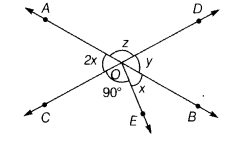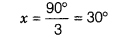# In the given figure, ZCOE = 90°. Find the value of x

In the given figure, ZCOE = 90°. Find the value of x.Here, ∠BOD =∠AOC

2 x = y

Now, ∠COE + ∠EOB + ∠AOC = 180°

90° + x + 2x = 180°

3x = 90°